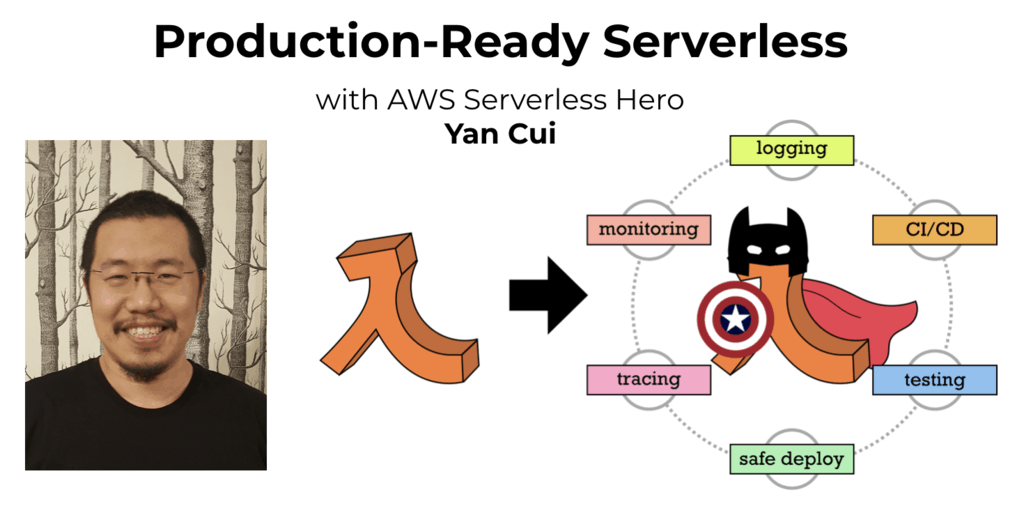# Project Euler – Problem 21 Solution

Check out my new course Learn you some Lambda best practice for great good! and learn the best practices for performance, cost, security, resilience, observability and scalability.

#### Problem

Let d(n) be defined as the sum of proper divisors of n (numbers less than n which divide evenly into n).

If d(a) = b and d(b) = a, where a != b, then a and b are an amicable pair and each of a and b are called amicable numbers.

For example, the proper divisors of 220 are 1, 2, 4, 5, 10, 11, 20, 22, 44, 55 and 110; therefore d(220) = 284. The proper divisors of 284 are 1, 2, 4, 71 and 142; so d(284) = 220.

Evaluate the sum of all the amicable numbers under 10000.

#### Solution

```open System

let findDivisors(n) =
let upperBound = int32(Math.Sqrt(double(n)))

[1..upperBound]
|> Seq.filter (fun x -> n % x = 0)
|> Seq.collect (fun x -> [x; n/x])
|> Seq.filter (fun x -> x <> n)

let d(n) = findDivisors(n) |> Seq.sum
let dList = [ for n = 1 to 9999 do yield (n, d(n)) ]

dList
|> List.filter (fun (a, da) -> dList
|> List.exists (fun (b, db) -> b = da && a = db && a <> b))
|> List.sumBy (fun (n, dn) -> n)
```

First I defined a findDivisors function which returns all the divisors of a given number excluding itself as is the case in the example given in the brief. Then I defined the function d which returns the sum of the given number’s proper divisors.

To get to the answer, I generated a list of tuples for the numbers from 1 to 9999 in the form of (n, d(n)) and from there I iterated through the list and for each tuple check whether there exists another tuple which matches the conditions required for the two to be considered an amicable pair.

You might have noticed in the function applied to List.filter to identify amicable pairs that I have decomposed the tuple into (a, da), as I have mentioned before this is a form of the pattern matching in F#. The same pattern matching technique is also used when I calculate the sum of the amicable pairs.Hi, I’m Yan. I’m an AWS Serverless Hero and the author of Production-Ready Serverless.

I specialise in rapidly transitioning teams to serverless and building production-ready services on AWS.

Are you struggling with serverless or need guidance on best practices? Do you want someone to review your architecture and help you avoid costly mistakes down the line? Whatever the case, I’m here to help.Check out my new course, Learn you some Lambda best practice for great good! In this course, you will learn best practices for working with AWS Lambda in terms of performance, cost, security, scalability, resilience and observability. Enrol now and enjoy a special preorder price of £9.99 (~\$13).Are you working with Serverless and looking for expert training to level-up your skills? Or are you looking for a solid foundation to start from? Look no further, register for my Production-Ready Serverless workshop to learn how to build production-grade Serverless applications!